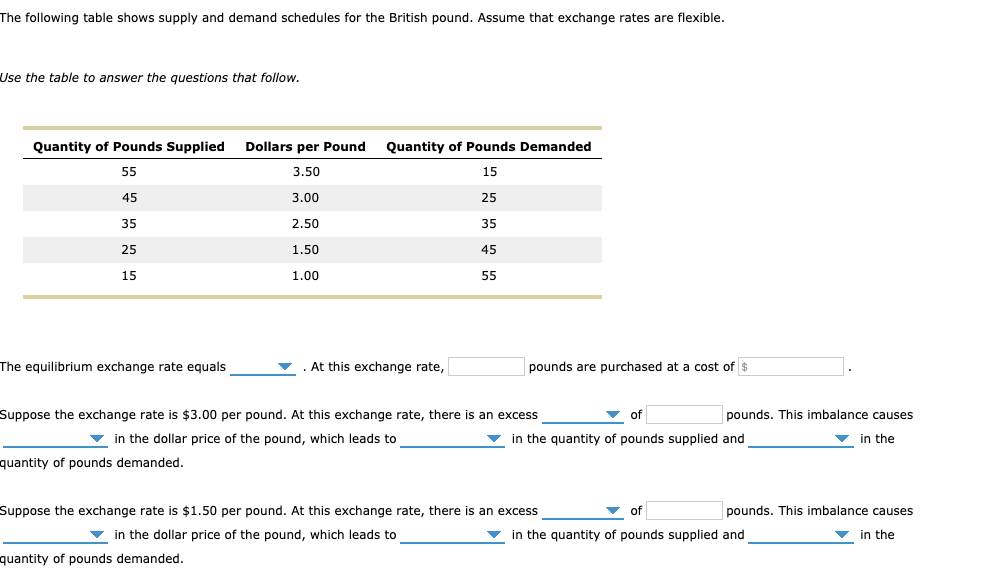### Create an Account

Home / Questions / The following table shows supply and demand schedules for the British pound Assume that ex...

# The following table shows supply and demand schedules for the British pound Assume that exchange rates are flexible. Use theThe following table shows supply and demand schedules for the British poundThe following table shows supply and demand schedules for the British pound. Assume that exchange rates are flexible. Use the table to answer the questions that follow. Quantity of Pounds Supplied Dollars per Pound Quantity of Pounds Demanded 55 3.50 15 45 3.00 25 35 2.50 35 25 1.50 45 15 1.00 55 At this exchange rate, pounds are purchased at a cost of \$ The equilibrium exchange rate equals Suppose the exchange rate is \$3.00 per pound. At this exchange rate, there is an excess of pounds. This imbalance causes in the quantity of pounds supplied and in the dollar price of the pound, which leads to in the quantity of pounds demanded Suppose the exchange rate is \$1.50 per pound. At this exchange rate, there is an excess of pounds. This imbalance causes in the dollar price of the pound, which leads to in the in the quantity of pounds supplied and quantity of pounds demanded.

Feb 06 2020 View more View LessGet Solution